•matlab
• ## R QQplot的demo和理解

千次阅读 2020-08-18 15:19:51
R QQplot的demo和理解 目录R QQplot的demo和理解N(0,1)正态分布图像二级目录 N(0,1)正态分布图像 set.seed(0) x <- rnorm(1000, mean = 0, sd = 1) par(mfrow = c(1, 2), pty = "s") qqPlot(x, main="QQ Plot") ...
R QQplot的demo和理解需要的library正态分布样例 (Samples for  𝑁(0,1))右偏分布样例 (Samples for right skewed distribution）左偏分布样例(Samples for left skewed distribution)短尾分布样例 (Samples for shot tailed distribution)总结写在最后
需要的library
library("car")
library(fGarch)

正态分布样例 (Samples for  𝑁(0,1))
set.seed(0)
x <- rnorm(1000, mean = 0, sd = 1)
par(mfrow = c(1, 2), pty = "s")
qqPlot(x, main="QQ Plot")
hist(x, n = 50, freq=FALSE, main="Distribution of Residuals", border = "white", col = "steelblue")
#xfit<-seq(min(x),max(x),length=50)
#yfit<-dnorm(xfit)
#lines(xfit, yfit, col = 'red', lwd = 3)可以看到上图qqplot图（左图）的点基本都躺在红色拟合线上，这种图像表明数据分布是近似正态分布。
右图为同数据生成的分布图，下同。
右偏分布样例 (Samples for right skewed distribution）
set.seed(0)
par(mfrow = c(1, 2), pty = "s")
snorm = rsnorm(1000, mean = 0, sd = 1, xi = 3)
qqPlot(snorm, main="QQ Plot")
#hist(snorm, Probability=True, main="Distribution of Residuals")
hist(snorm, n = 25, probability = TRUE, border = "white", col = "steelblue")
#xfit<-seq(min(snorm),max(snorm),length=50)
#yfit<-dnorm(xfit)
#lines(xfit, yfit, col = 'red', lwd = 3)上图qqplot图红色拟合线的起点和终点都位于对角线（y=x）下方，或者说右方，则这种qqplot图像表示数据分布为右偏分布。
左偏分布样例(Samples for left skewed distribution)
set.seed(0)
par(mfrow = c(1, 2), pty = "s")
snorm = rsnorm(1000, mean = 0, sd = 1, xi = -3)
qqPlot(snorm, main="QQ Plot")
hist(snorm, n=50, freq=FALSE, main="Distribution of Residuals",  border = "white", col = "steelblue")
#xfit<-seq(min(snorm),max(snorm),length=50)
#yfit<-dnorm(xfit)
#lines(xfit, yfit, col = 'red', lwd = 3)上图qqplot图红色拟合线的起点和终点都位于对角线（y=x）上方，或者说左方，则这种qqplot图像表示数据分布为左偏分布。
短尾分布样例 (Samples for shot tailed distribution)
set.seed(0)
par(mfrow = c(1, 2), pty = "s")
short = runif(1000,min=0,max=2)
qqPlot(short, main="QQ Plot")
hist(short, n=100, freq=FALSE, main="Distribution of Residuals",  border = "white", col = "steelblue")set.seed(0)
par(mfrow = c(1, 2), pty = "s")
long <- rcauchy(1000, location = 0, scale=1)
qqPlot(long, main="QQ Plot")
hist(long, n=100, freq=FALSE, main="Distribution of Residuals",  border = "white", col = "steelblue")
#xfit<-seq(min(long),max(long),length=100)
#yfit<-dcauchy(xfit)
#lines(xfit, yfit, col = 'red', lwd = 3)由以上2个样例可以看出， 当qqplot图的拟合线与对角线（y=x）有交叉时，且该拟合线比较接近水平或竖直时，则这种qqplot图像表明数据是分布是短尾分布。
总结
qqplot 是一种较为便捷的方法来判断数据分布是怎么样的。
那么就会有人问，直接拿hist()看分布不香吗？
我是同意上面这种想法的。
我理解qqplot核心是用来比较两组数据分布是否类似。
什么意思？
将上面的例子换个方式思考，你就会明白。
前面举的例子都是用我们创造的一组数据跟正态分布的数据进行比较。
换句话说，就是我们创造的这组数据的分布跟正态分布数据的分布是不是一样的。
如果把正态分布的数据换成其他的数据，不就成了比较A，B两组数据的分布是否是一样的了嘛~
par(mfrow = c(5, 2))
set.seed(0)

x <- rnorm(1000, mean = 0, sd = 1)
qqPlot(x, main="QQ Plot")
hist(x, n = 25, freq=FALSE, main="Distribution of Residuals", border = "white", col = "steelblue")

snorm = rsnorm(1000, mean = 0, sd = 1, xi = 3)
qqPlot(snorm, main="QQ Plot")
hist(snorm, n = 25, probability = TRUE, border = "white", col = "steelblue")

snorm = rsnorm(1000, mean = 0, sd = 1, xi = -3)
qqPlot(snorm, main="QQ Plot")
hist(snorm, n = 25, probability = TRUE, border = "white", col = "steelblue")

short = runif(1000,min=0,max=2)
qqPlot(short, main="QQ Plot")
hist(short, n=25, freq=FALSE, main="Distribution of Residuals",  border = "white", col = "steelblue")

long <- rcauchy(1000, location = 0, scale=0.5)
qqPlot(long, main="QQ Plot")
hist(long, n=100, freq=FALSE, main="Distribution of Residuals",  border = "white", col = "steelblue")写在最后
如果您有疑问或其他的思考，欢迎给我留言或评论，如果以上表述有错误的地方，也欢迎并感谢指出。


展开全文r语言 统计学
• QQPlot用于直观验证一组数据是否来自某个分布，或者验证某两组数据是否来自同一（族）分布。在教学和软件中常用的是检验数据是否来自于正态分布。 详细信息参考： ... -------------------------------------------...
QQPlot用于直观验证一组数据是否来自某个分布，或者验证某两组数据是否来自同一（族）分布。在教学和软件中常用的是检验数据是否来自于正态分布。
详细信息参考：
-----------------------------------------------------------------
（原文如下）
Quantile-Quantile
(q-q) Plots

Author(s)
David Scott

Prerequisites
Histograms, Distributions, Percentiles, Describing
Bivariate Data, Normal
Distributions Learning Objectives
State what q-q plots are used for.Describe the shape of a q-q plot when the distributional assumption is met.Be able to create a normal q-q plot.
Introduction
The quantile-quantile or q-q plot is an exploratory graphical device used to check the validity of a distributional assumption for a data set. In general, the basic idea is to compute the theoretically expected value for each data point based on the distribution
in question. If the data indeed follow the assumed distribution, then the points on the q-q plot will fall approximately on a straight line.

Before delving into the details of q-q plots, we first describe two related graphical methods for assessing distributional assumptions: the histogram and the cumulative distribution function (CDF). As will be seen, q-q plots are more general than these alternatives.

Assessing Distributional Assumptions
As an example, consider data measured from a physical device such as the spinner depicted in Figure 1. The red arrow is spun around the center, and when the arrow stops spinning, the number between 0 and 1 is recorded. Can we determine if the spinner is fair?Figure 1. A physical device that gives samples from a uniform distribution.

If the spinner is fair, then these numbers should follow a uniform distribution. To investigate whether the spinner is fair, spin the arrow n times, and record the measurements by {μ1, μ2, ..., μn}. In this example, we collect
n = 100 samples. The histogram provides a useful visualization of these data. In Figure 2, we display three different histograms on a probability scale. The histogram should be flat for a uniform sample, but the visual perception varies depending on whether
the histogram has 10, 5, or 3 bins. The last histogram looks flat, but the other two histograms are not obviously flat. It is not clear which histogram we should base our conclusion on.Figure 2. Three histograms of a sample of 100 uniform points.

Alternatively, we might use the cumulative distribution function (CDF), which is denoted by F(μ). The CDF gives the probability that the spinner gives a value less than or equal to μ, that is, the probability that the red arrow lands in the interval [0, μ].
By simple arithmetic, F(μ) = μ, which is the diagonal straight line y = x. The CDF based upon the sample data is called the empirical CDF (ECDF), is denoted by,
and is defined to be the fraction of the data less than or equal to μ; that is,In general, the ECDF takes on a ragged staircase appearance.
For the spinner sample analyzed in Figure 2, we computed the ECDF and CDF, which are displayed in Figure 3. In the left frame, the ECDF appears close to the line y = x, shown in the middle frame. In the right frame, we overlay these two curves and verify that
they are indeed quite close to each other. Observe that we do not need to specify the number of bins as with the histogram.Figure 3. The empirical and theoretical cumulative distribution functions of a sample of 100 uniform points.

q-q plot for uniform data

The q-q plot for uniform data is very similar to the empirical CDF graphic, except with the axes reversed. The q-q plot provides a visual comparison of the sample quantiles to the corresponding theoretical quantiles. In general, if the points in a q-q plot
depart from a straight line, then the assumed distribution is called into question.

Here we define the qth quantile of a batch of n numbers as a number ξqsuch that a fraction q x n of the sample is less than ξq, while a fraction (1 - q) x n of the sample is greater than ξq. The best known quantile is the median,
ξ0.5, which is located in the middle of the sample.

Consider a small sample of 5 numbers from the spinner: μ1 = 0.41, μ2 =0.24, μ3 =0.59, μ4 =0.03,and μ5 =0.67.
Based upon our description of the spinner, we expect a uniform distribution to model these data. If the sample data were “perfect,” then on average there would be an observation in the middle of each of the 5 intervals: 0 to .2, .2 to .4, .4 to .6, and so on.
Table 1 shows the 5 data points (sorted in ascending order) and the theoretically expected value of each based on the assumption that the distribution is uniform (the middle of the interval).

Table 1. Computing the Expected Quantile Values.

Data (μ)

Rank (i)

Middle of the
ith Interval

.03
.24
.41
.59
.67

1
2
3
4
5

.1
.3
.5
.7
.9

The theoretical and empirical CDFs are shown in Figure 4 and the q-q plot is shown in the left frame of Figure 5.Figure 4. The theoretical and empirical CDFs of a small sample of 5 uniform points, together with the expected values of the 5 points (red dots in the right frame).

In general, we consider the full set of sample quantiles to be the sorted data values

μ(1) < μ(2) < μ(3) < ··· < μ(n-1) < μ(n) ,

where the parentheses in the subscript indicate the data have been ordered. Roughly speaking, we expect the first ordered value to be in the middle of the interval (0, 1/n), the second to be in the middle of the interval (1/n, 2/n), and the last to be in the
middle of the interval ((n - 1)/n, 1). Thus, we take as the theoretical quantile the valuewhere q corresponds to the ith ordered sample value. We subtract the quantity 0.5 so that we are exactly in the middle of the interval ((i - 1)/n, i/n). These ideas are depicted in the right frame of Figure 4 for our small sample of size n = 5.

We are now prepared to define the q-q plot precisely. First, we compute the n expected values of the data, which we pair with the n data points sorted in ascending order. For the uniform density, the q-q plot is composed of the n ordered pairsThis definition is slightly different from the ECDF, which includes the points (u(i), i/n). In the left frame of Figure 5, we display the q-q plot of the 5 points in Table 1. In the right two frames of Figure 5, we display the q-q plot of the same
batch of numbers used in Figure 2. In the final frame, we add the diagonal line y = x as a point of reference.Figure 5. (Left) q-q plot of the 5 uniform points. (Right) q-q plot of a sample of 100 uniform points.

The sample size should be taken into account when judging how close the q-q plot is to the straight line. We show two other uniform samples of size n = 10 and n = 1000 in Figure 6. Observe that the q-q plot when n = 1000 is almost identical to the line y =
x, while such is not the case when the sample size is only n = 10.Figure 6. q-q plots of a sample of 10 and 1000 uniform points.

In Figure 7, we show the q-q plots of two random samples that are not uniform. In both examples, the sample quantiles match the theoretical quantiles only at the median and at the extremes. Both samples seem to be symmetric around the median. But the data in
the left frame are closer to the median than would be expected if the data were uniform. The data in the right frame are further from the median than would be expected if the data were uniform.Figure 7. q-q plots of two samples of size 1000 that are not uniform.

In fact, the data were generated in the R language from beta distributions with parameters a = b = 3 on the left and a = b =0.4 on the right. In Figure 8 we display histograms of these two data sets, which serve to clarify the true shapes of the densities.
These are clearly non-uniform.Figure 8. Histograms of the two non-uniform data sets.

q-q plot for normal data

The definition of the q-q plot may be extended to any continuous density. The q-q plot will be close to a straight line if the assumed density is correct. Because the cumulative distribution function of the uniform density was a straight line, the q-q plot
was very easy to construct. For data that are not uniform, the theoretical quantiles must be computed in a different manner.

Let {z1, z2, ..., zn} denote a random sample from a normal distribution
with mean μ = 0 and standard deviation σ = 1. Let the ordered values be
denoted by

z{1) < z(2) < z(3) < ... < z(n-1) <z(n).

These n ordered values will play the role of the sample quantiles.

Let us consider a sample of 5 values from a distribution to see how they compare with what would be expected for a normal distribution. The 5 values in ascending order are shown in the first column of Table 2.

Table 2. Computing the expected quantile values for normal data.

Data (z)

Rank (i)

Middle of the
ith Interval

Normal(z)

-1.96
-.78
.31
1.15
1.62

1
2
3
4
5

.1
.3
.5
.7
.9

-1.28
-0.52
0.00
0.52
1.28

Just as in the case of the uniform distribution, we have 5 intervals. However, with a normal distribution the theoretical quantile is not the middle of the interval but rather the inverse of the normal distribution for the middle of the interval. Taking the
first interval as an example, we want to know the z value such that 0.1 of the area in the normal distribution is below z. This can be computed using the Inverse Normal Calculator as shown in Figure 9. Simply set the “Shaded Area” field to the middle of the
interval (0.1) and click on the “Below” button. The result is -1.28. Therefore, 10% of the distribution is below a z value of -1.28.Figure 9. Example of the Inverse Normal Calculator for finding a value of the expected quantile from a normal distribution.

The q-q plot for the data in Table 2 is shown in the left frame of Figure 11.

In general, what should we take as the corresponding theoretical quantiles? Let the cumulative distribution function of the normal density be denoted by Φ(z). In the previous example, Φ(-1.28) = 0.10 and Φ(0.00) = 0.50. Using the quantile notation, if ξq is
the qth quantile of a normal distribution, then

Φ(ξq)= q.

That is, the probability a normal sample is less than ξq is in fact just q.

Consider the first ordered value, z(1). What might we expect the value of Φ(z(1)) to be? Intuitively, we expect this probability to take on a value in the interval (0, 1/n). Likewise, we expect Φ(z(2)) to take on a value in
the interval (1/n, 2/n). Continuing, we expect Φ(z(n)) to fall in the interval ((n - 1)/n, 1). Thus, the theoretical quantile we desire is defined by the inverse (not reciprocal) of the normal CDF. In particular, the theoretical quantile corresponding
to the empirical quantile z(i) should befor i = 1, 2, ..., n.

The empirical CDF and theoretical quantile construction for the small sample given in Table 2 are displayed in Figure 10. For the larger sample of size 100, the first few expected quantiles are -2.576, -2.170, and -1.960.Figure 10. The empirical CDF of a small sample of 5 normal points, together with the expected values of the 5 points (red dots in the right frame).

In the left frame of Figure 11, we display the q-q plot of the small normal sample given in Table 2. The remaining frames in Figure 11 display the q-q plots of normal random samples of size n = 100 and n = 1000. As the sample size increases, the points in the
q-q plots lie closer to the line y = x.Figure 11. q-q plots of normal data.

As before, a normal q-q plot can indicate departures from normality. The two most common examples are skewed data and data with heavy tails (large kurtosis). In Figure 12, we show normal q-q plots for a chi-squared (skewed) data set and a Student’s-t (kurtotic)
data set, both of size n = 1000. The data were first standardized. The red line is again y = x. Notice, in particular, that the data from the t distribution follow the normal curve fairly closely until the last dozen or so points on each extreme.Figure 12. q-q plots for standardized non-normal data (n = 1000).

q-q plots for normal data with general mean and scale

Our previous discussion of q-q plots for normal data all assumed that our data were standardized. One approach to constructing q-q plots is to first standardize the data and then proceed as described previously. An alternative is to construct the plot directly
from raw data.

In this section, we present a general approach for data that are not standardized. Why did we standardize the data in Figure 12? The q-q plot is comprised of the n pointsIf the original data {zi} are normal, but have an arbitrary mean μ and standard deviation σ, then the line y = x will not match the expected theoretical quantiles. Clearly, the linear transformation

μ + σ ξq

would provide the qth theoretical quantile on the transformed scale. In practice, with a new data set

{x1,x2,...,xn} ,

the normal q-q plot would consist of the n pointsInstead of plotting the line y = x as a reference line, the line

y = M + s · x

should be composed, where M and s are the sample moments (mean and standard deviation) corresponding to the theoretical moments μ and σ. Alternatively, if the data are standardized, then the line y = x would be appropriate, since now the sample mean would be
0 and the sample standard deviation would be 1.

Example: SAT Case Study

The SAT case study followed the academic achievements of 105 college students majoring in computer science. The first variable is their verbal SAT score and the second is their grade point average (GPA) at the university level. Before we compute inferential
statistics using these variables, we should check if their distributions are normal. In Figure 13, we display the q-q plots of the verbal SAT and university GPA variables.Figure 13. q-q plots for the student data (n = 105).

The verbal SAT seems to follow a normal distribution reasonably well, except in the extreme tails. However, the university GPA variable is highly non-normal. Compare the GPA q-q plot to the simulation in the right frame of Figure 7. These figures are very similar,
except for the region where x ≈ -1. To follow these ideas, we computed histograms of the variables and their scatter diagram in Figure 14. These figures tell quite a different story. The university GPA is bimodal, with about 20% of the students falling into
a separate cluster with a grade of C. The scatter diagram is quite unusual. While the students in this cluster all have below average verbal SAT scores, there are as many students with low SAT scores whose GPAs were quite respectable. We might speculate as
to the cause(s): different distractions, different study habits, but it would only be speculation. But observe that the raw correlation between verbal SAT and GPA is a rather high 0.65, but when we exclude the cluster, the correlation for the remaining 86
students falls a little to 0.59.Figure 14. Histograms and scatter diagram of the verbal SAT and GPA variables for the 105 students.

Discussion

Parametric modeling usually involves making assumptions about the shape of data, or the shape of residuals from a regression fit. Verifying such assumptions can take many forms, but an exploration of the shape using histograms and q-q plots is very effective.
The q-q plot does not have any design parameters such as the number of bins for a histogram.

In an advanced treatment, the q-q plot can be used to formally test the null hypothesis that the data are normal. This is done by computing the correlation coefficient of the n points in the q-q plot. Depending upon n, the null hypothesis is rejected if the
correlation coefficient is less than a threshold. The threshold is already quite close to 0.95 for modest sample sizes.

We have seen that the q-q plot for uniform data is very closely related to the empirical cumulative distribution function. For general density functions, the so-called probability integral transform takes a random variable X and maps it to the interval (0,
1) through the CDF of X itself, that is,

Y = FX(X)

which has been shown to be a uniform density. This explains why the q-q plot on standardized data is always close to the line y = x when the model is correct.
Finally, scientists have used special graph paper for years to make relationships linear (straight lines). The most common example used to be semi-log paper, on which points following the formula y = aebx appear linear. This follows of course
since log(y) = log(a) + bx, which is the equation for a straight line. The q-q plots may be thought of as being “probability graph paper” that makes a plot of the ordered data values into a straight line. Every density has its own special probability graph
paper.

展开全文plot
• 最近读文章遇到qqplot的问题，看了几个视频讲解，大致有了个了解 首先我们需要了解什么是 quantile : 从这个视频里有个大概了解：https://www.youtube.com/watch?v=IFKQLDmRK0Y quantile – median – it ...
最近读文章遇到qqplot的问题，看了几个视频讲解，大致有了个了解

首先我们需要了解什么是 quantile :

quantile – median – it splits the data into equal sized groups. 50% quantile

如果我们将数据分为4个大小相等的组，则25％的分位数表示25％的数据点小于它。

Quantile 就是把样本区分成相同大小的组分.

我们也可以根据样本来划分quantile，见下面的图例：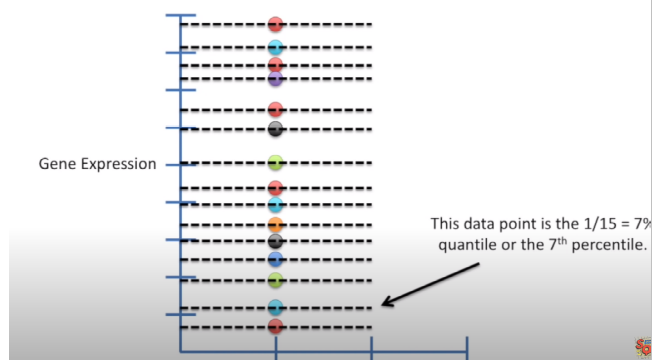在R中，分位数函数有9种计算方法，如果您的数据集很大，那么所有方法都将得出非常相似的结果，数据集合小则反之。

第二，什么是正态分布
正态分布（也称为高斯分布）是关于均值对称的概率分布，这表明均值附近的数据比不均值的数据更频繁地出现。 在图形形式中，正态分布将显示为钟形曲线。
-例如以下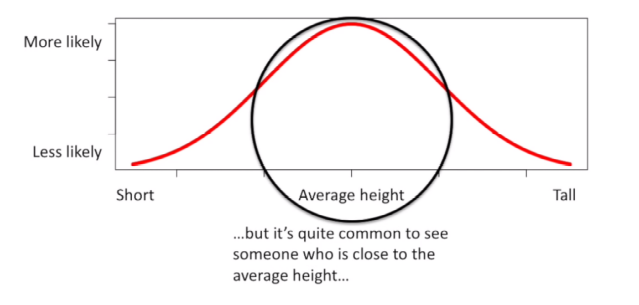x

根据正态分布的特点我们知道均值+/-2个标准差之间代表了95%的数据，这个例子左侧是婴儿身高分布，右边是成人身高分布，它们都是正态分布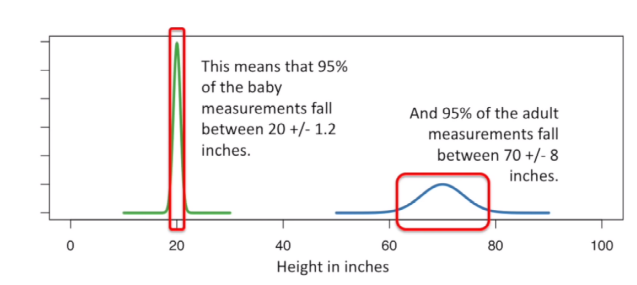下面讲下什么是qq plot - 全称是quantile-quantile plot，可以用来检测一个分布是否符合正态分布、均匀分布.... https://www.youtube.com/watch?v=X9_ISJ0YpGw

比如我们有一组数字 3.89 3.99 4.5 6.7 6.8 8.7 9.5 10.2 12.5 （9个数字）检测是否符合正态分布

根据我们上面的定义 3.89对应的是1/10 quantile，3.4对应的第二个1/10quantile，我们把正态分布均分成10等分，得到下图,对应1/10的z score是-1.28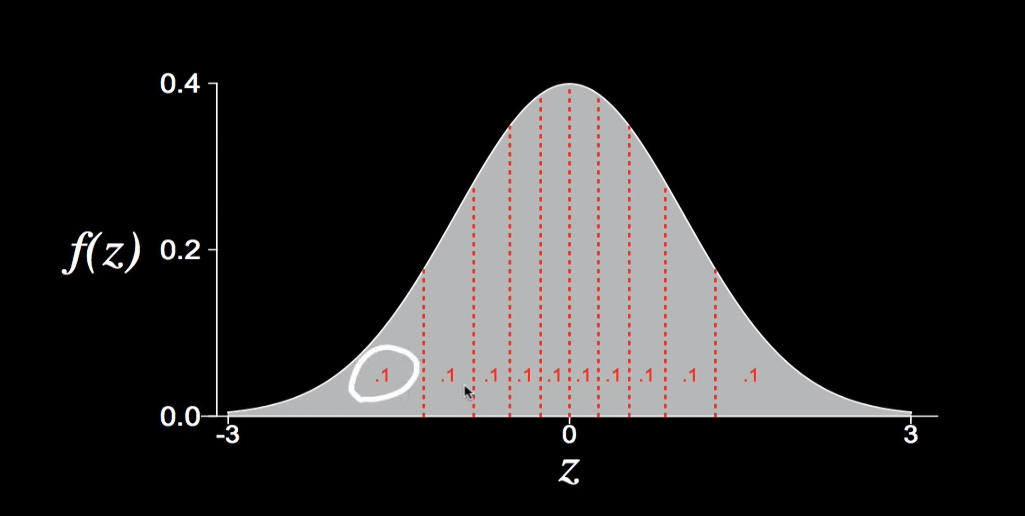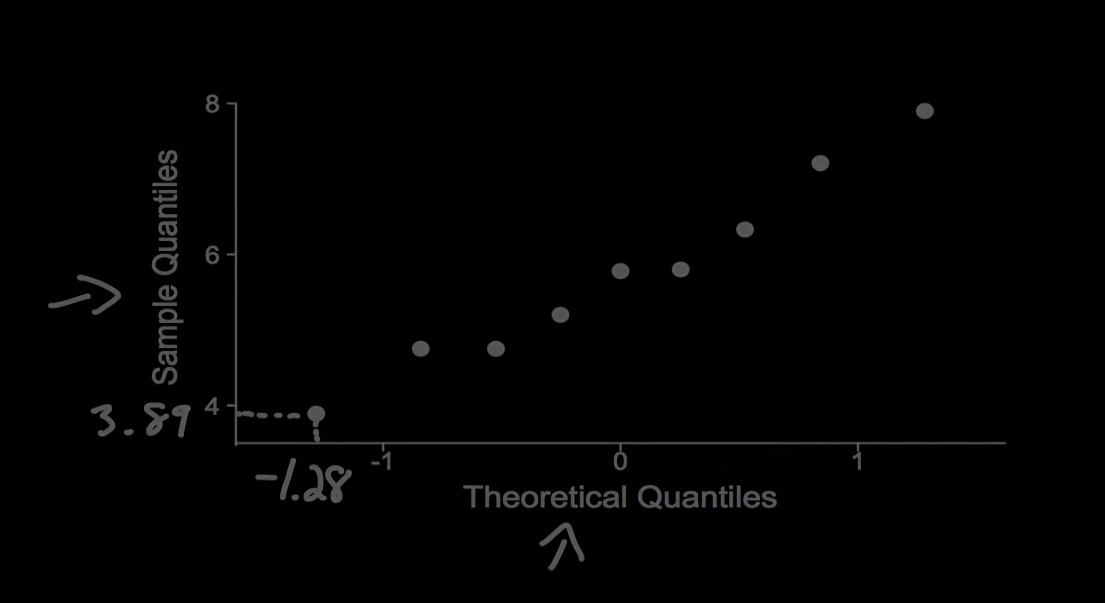把对应的z score填写为x轴，对应的实际轴为y轴就可以得到qqplot图

如果大多数的点都在线上，就可以说明大致符合正态分布

qqplot的x y轴可以互换，下面是一些例子

比如这种负偏分布，与划线的正态分布相比，我们在小的值里，实际值并不能得到比理论分布那么小的值，因为它没有尾巴，很快就停止了，相反在大的值里得到比我们期望的更大的值，因为尾巴更长。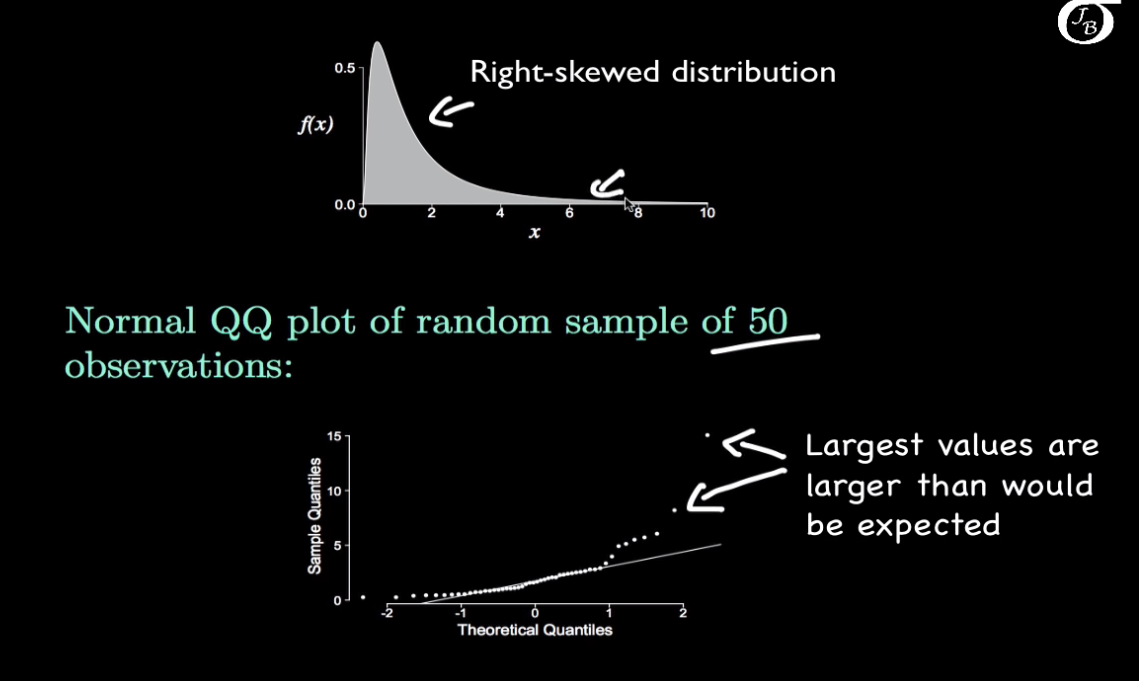另外，qqplot不仅仅可以用于正态分布检验，也可以用于uniform 等其他分布的检验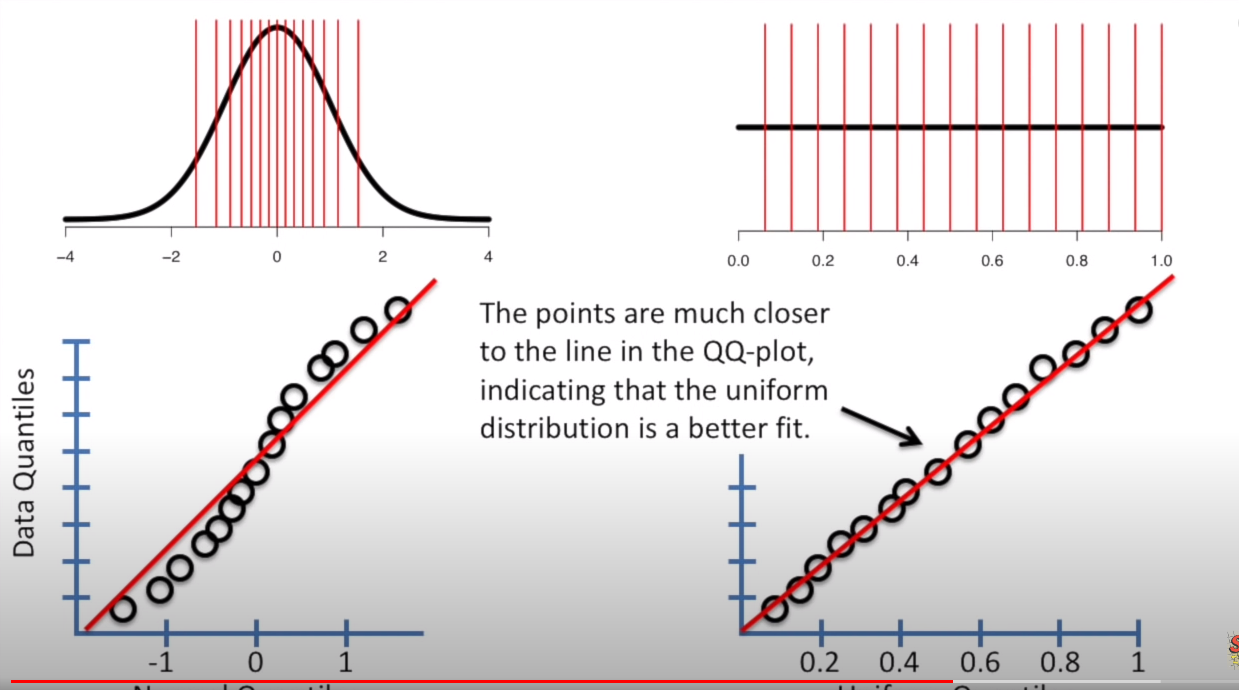参考资料


展开全文• ## ggplot2做qqplot图

千次阅读 2015-05-12 21:46:00
转载自http://stats.stackexchange.com/questions/12392/how-to-compare-two-datasets-with-q-q-plot-using-ggplot2 ...ggplot2提供了一个qqplot的函数，但这个函数并不能对两组观测的数字进行作图。...
转载自http://stats.stackexchange.com/questions/12392/how-to-compare-two-datasets-with-q-q-plot-using-ggplot2
感谢csgillespie的答案

qqplot是可以直观反应两组数字是否属于同一分布的作图。ggplot2提供了一个qqplot的函数，但这个函数并不能对两组观测的数字进行作图。与此相对的是，R中却有原生函数qqplot来提供这个作图。
以下是如何利用qqplot函数的方法，使用ggplot来作图。
这是R中qqplot的原始方法：

R> qqplot
function (x, y, plot.it = TRUE, xlab = deparse(substitute(x)),
ylab = deparse(substitute(y)), ...)
{
sx <- sort(x)
sy <- sort(y)
lenx <- length(sx)
leny <- length(sy)
if (leny < lenx)
sx <- approx(1L:lenx, sx, n = leny)$y if (leny > lenx) sy <- approx(1L:leny, sy, n = lenx)$y
if (plot.it)
plot(sx, sy, xlab = xlab, ylab = ylab, ...)
invisible(list(x = sx, y = sy))
}
<environment: namespace:stats>

这是ggplot利用同样方法进行作图的代码：

x <- rnorm(10);y <- rnorm(20)

sx <- sort(x); sy <- sort(y)
lenx <- length(sx)
leny <- length(sy)
if (leny < lenx)sx <- approx(1L:lenx, sx, n = leny)$y if (leny > lenx)sy <- approx(1L:leny, sy, n = lenx)$y

require(ggplot2)
g = ggplot() + geom_point(aes(x=sx, y=sy))
g

转载于:https://www.cnblogs.com/yumtaoist/p/4498729.html
展开全文• 在GWAS研究中，Manhattan plotQQ plot是最常画的两类图，它们可以把跟研究的性状（比如，基因型和身高）显著相关的基因位点清晰地展现出来，不少读者朋友应该都懂得如何画这样的图，但我想应该不是每个人都能够...
• QQplot 横坐标表示的是属性的其中一个测量值1，纵坐标表示另一个测量值2。散点是分位点。点的横纵坐标是这个测量值1和测量值2的分位点的取值。 from scipy import stats from matplotlib import pyplot as ...
• ## R QQplot

千次阅读 2013-12-30 15:24:50
部分借鉴 百度百科及...QQ图的作用 用于直观验证一组数据是否来自某个分布，或者验证某两组数据是否来自同一（族）分布。在教学和软件中常用的是检验数据是否来自
• The QQ plot shows the expected distribution of association test statistics (X-axis) across the million SNPs compared to the observed values (Y-axis). Any deviation from the X=Y line implies a consis
• 画曼哈顿图和QQ plot 首推R包“qqman”，简约方便。下面具体介绍以下。 一、画曼哈顿图 install.packages("qqman") library(qqman) 　 1、准备包含SNP, CHR, BP, P的文件gwasResults（如果没有zscore...
• import tushare as ts import pandas as pd import numpy as np import matplotlib.pyplot as plt from scipy import stats sh=ts.get_hist_data('sh') ...stats.probplot(sh['close'],dist='norm',plot=plt) ...
• 文章目录 任务 解决方案 任务 要使用某个模型，而这个模型的假设之一是数据服从正太分布。...qqplot(ph) %QQ图上看，ph的分位数跟标准正态分布的分位数并非线性，印证了ph不接近正态分布matlab
• 1. 简单实现qq图 输入为一个vector，我们以a <- seq(1, 250, 1)做为示例数据 a<-seq(1,250,1) 利用qqnorm函数直接绘制出了如下正态检验qq图 qqnorm(a) 还可以进一步使用qqline命令在qq图上...
• 关于QQ-plot的一点个人见解 去年考到了QQ plot，因为错的十分惨烈，到现在都对当时对答案时的场景印象深刻。但是直到半年后的现在才大概明白QQ plot，该打。 简要概述：QQ plot（quantile-quantile plot），也称...数据分析 大数据
• 这几天看了一下QQ-plot以及在Matlab中的实现，可是Matlab自带的qqplot函数不能满足我的使用，因此在网上搜索到了一个好工具：gqqplot。gqqplot可以于很多常见的分布进行比较，而qqplot仅仅可以比较正态分布。其zip...
• ## 如何画QQ-plot

千次阅读 2020-03-24 18:45:09
看到QQ-plot，首先要注意的就是这个QQ和那个大家熟知的QQ不是一回事！ 那接下来我们看看这个QQ图到底是啥呢？ 先上图： 根据Wiki的定义： 在统计学中，QQ图（Q代表分位数Quantile）是一种通过比较两个概率...机器学习
• import matplotlib.pyplot as plt from numpy.random import randn plt.style.use('ggplot') plot_data1=randn(50).cumsum() plot_data2=randn(50).cumsum() plot_data3=randn(50).cumsum...plot_data4=randn(50).c...matplotlib
• QQ-plot深入理解与实现 26JUN June 26, 2013 最近在看关于CSI(Channel State Information)相关的论文，发现论文中用到了QQ-plot。Sigh！我承认我是第一次见到这个名词，异常陌生。维基百科给出了如下定义：...
• ax.boxplot(box_plot_data,notch=False,sym='.',vert=True,whis=1.5,showmeans=True,labels=box_labels) ax.xaxis.set_ticks_position('bottom') ax.yaxis.set_ticks_position('left') ax.set_title('Box Plot:......